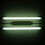## Monday, August 08, 2005

### Favorite Theorems: Abstract Complexity

July Edition

As a graduate student, Manuel Blum wanted to study computational complexity freed from any specific machine model. His paper set the tone for much of complexity of the late 60's.

Manuel Blum, A Machine-Independent Theory of the Complexity of Recursive Functions, JACM 1967.

Blum defined a resource measure as any partially computable function ΦM (think time) of a machine M that fulfilled some simple axioms.

1. For all x, M(x) halts iff ΦM(x) halts.
2. The language {<M,x,m> | ΦM(x)=m} is computable.
Blum argues that time complexity on any reasonable model of Turing machine would fulfill these axioms. He also noticed that space complexity also fulfills the axioms. Though the axioms are very general, Blum shows their power with the speed-up theorem.

Speed-Up Theorem: For every computable function r, there is a language L such that if M accepts L there is an N accepting L with r(x,ΦN(x))≤ΦM(x) for almost all x.

For example there is some language L such that if any algorithm computes L in t(n) steps there is another algorithm computing L in log t(n) steps. This might seem to violate the time hierarchy but t(n) does not have the time constructibility needed for the hierarchy. Blum also showed there was a language that couldn't be sped up much and a computably-bounded relationship between any two abstract resource measures.

Borodin and Trakhtenbrot independently proved the gap theorem for abstract complexity measures.

Gap Theorem: Given any computable function g(x)≥x there is a recursive function t(x) such that if ΦM(x)≤g(t(x)) for all x then there is an N accepting the same language as M with ΦN(x)≤t(x) for almost all x.

In particular there is a function t(n) such that DTIME(t(n))=DTIME(2t(n)) and thus DTIME(t(n))=DSPACE(t(n)).

McCreight and Meyer proved the union theorem.

Union Theorem: Let t1, t2, … be a computable enumeration of increasing resource bounds. There is a computable function t such that the following are equivalent for all M.

• For some i and almost all x, ΦM(x)≤ti(x).
• For almost all x, &PhiM(x)≤t(x).
For example there are computable functions t1 and t2 such that DTIME(t1(n)) is equal to P and DTIME(t2(n)) is exactly the primitive recursive languages.

McCreight and Meyer also give an honesty theorem showing that computable t there is (in a weak sense) a time-constructible t' such that languages computable with resource bound t are equal to languages computable with resource bound t'.

After the P versus NP problem was popularized by Cook and Karp in 1971, the focus of complexity went to polynomial-time (which also was machine independent) and away from abstract complexity.

1.I view the SPEEDUP theorem as saying that
the attempt to assign to every decidable
set a complexity is impossible.
That is, there are WEIRD sets for which
we cannot intelligently assign a
complexity
(e.g., there is a set A such that if
YOU say A is in DTIME(T(n)) then I
can take take that algorithm and produce
one that shows A in DTIME(log(T(n)) ).
They are like non-measureable sets of reals
which cannot be assigned a measure.

2.The deemphasis on abstract complexity including speedup is unfortunate. For instance, three of the five problems with a monotone-nonmonotone gap (BOOLEAN MM, PERFECT MATCHING, and Tardos' CLIQUE-LIKE problem) rely upon MATRIX MULTIPLICATION, and Coppersmith and Winograd proved that no finite set of Strassen-type identities can achieve the infimum omega of all possible exponents of MM. That is, no algorithm for MM based on self-reduction can be (x^{1+epsilon})-optimal for all epsilon>0 under Blum's definition of optimality. The same holds true in Cohn et al's group theoretic framework for MM. Of the remaining two problems with a gap, BOOLEAN CONVOLUTION is conjectured by Schnorr and Stumpf not to have an optimal algorithm, and BOOLEAN SORTING provably has an optimal algorithm, but only a tiny log n gap. Note that HAMILTON CIRCUIT has an optimal algorithm by Levin's construction, as it can be verified in linear time. Therefore, the conjecture that "no problem with an \Omega(n^k) gap has an optimal algorithm" implies P!=NP, since Alon and Boppana showed that HAMILTON CIRCUIT has exponential monotone circuit complexity. If P!=NP, then all NP-complete problems have an optimal algorithm by Levin's construction. This type of argument does not relativize (too powerful an oracle eliminates the phenomena of speedup because an exhaustive search of all identities is considered uniform) nor naturalize (the proof of the existence of a faster algorithm is nonconstructive).

3.Umm guys... have you seenmy proof;

Regards,

Martin Musatov

4.5.6.